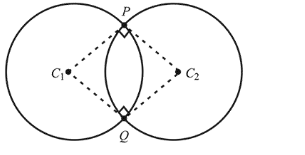# Let C1 and C2 be the centres of the circles

Question:

Let $\mathrm{C}_{1}$ and $\mathrm{C}_{2}$ be the centres of the circles $\mathrm{x}^{2}+\mathrm{y}^{2}-2 \mathrm{x}-2 \mathrm{y}-2=$ 0 and $x^{2}+y^{2}-6 x-6 y+14=0$ respectively. If $P$ and $Q$ are the points of intersection of these circles then, the area (in sq. units) of the quadrilateral $\mathrm{PC}_{1} \mathrm{QC}_{2}$ is :

1. (1) 8

2. (2) 6

3. (3) 9

4. (4) 4

Correct Option: , 4

Solution:$2 g_{1} g_{2}+2 f_{1} f_{2}=2(-1)(-3)+2(-1)(-3)=12$

$c_{1}+c_{2}=14-2=12$

Since, $2 g_{1} g_{2}+2 f_{1} f_{2}=c_{1}+c_{2}$

Hence, circles intersect orthogonally

$\therefore \quad$ Area of the quadrilateral $\mathrm{PC}_{1} \mathrm{QC}_{1}$

$=2\left(\frac{1}{2}\left(C_{1} P\right)\left(C_{2} P\right)\right)$

$=2 \times \frac{1}{2} r_{1} r_{2}=(2)(2)=4 \mathrm{sq}$, units